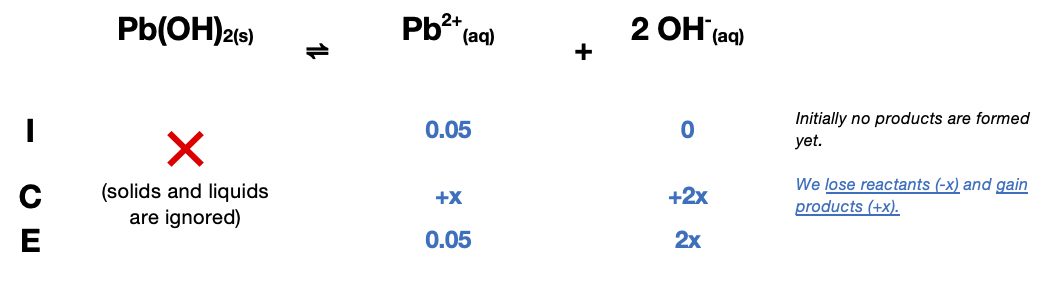# Problem: How many grams of Pb(OH)2 will dissolve in 500 mL of a 0.050-M PbCl2 solution (Ksp = 1.2 × 10–15)?

###### FREE Expert Solution

The ICE table for the dissociation of Pb(OH)2 is:The Ksp expression for Pb(OH)2 is:

${\mathbf{K}}_{\mathbf{sp}}\mathbf{=}\frac{\mathbf{products}}{\overline{)\mathbf{reactants}}}\mathbf{=}\left[{\mathrm{Pb}}^{2+}\right]{\left[{\mathrm{OH}}^{-}\right]}^{\mathbf{2}}$

Plugging in the value for Ksp (1.2 × 10–15) and equilibrium concentrations:

$\mathbf{1}\mathbf{.}\mathbf{2}\mathbf{×}{\mathbf{10}}^{\mathbf{-}\mathbf{15}}\mathbf{=}\mathbf{\left[}\mathbf{0}\mathbf{.}\mathbf{05}\mathbf{+}\mathbf{x}\mathbf{\right]}{\mathbf{\left[}\mathbf{2}\mathbf{x}\mathbf{\right]}}^{\mathbf{2}}$

Since the value of Ksp is very small, we can remove +x from the equation to simplify it:

$\mathbf{1}\mathbf{.}\mathbf{2}\mathbf{×}{\mathbf{10}}^{\mathbf{-}\mathbf{15}}\mathbf{=}\mathbf{\left[}\mathbf{0}\mathbf{.}\mathbf{05}\overline{)\mathbf{+}\mathbf{x}}\mathbf{\right]}{\mathbf{\left[}\mathbf{2}\mathbf{x}\mathbf{\right]}}^{\mathbf{2}}\phantom{\rule{0ex}{0ex}}\phantom{\rule{0ex}{0ex}}\mathbf{1}\mathbf{.}\mathbf{2}\mathbf{×}{\mathbf{10}}^{\mathbf{-}\mathbf{15}}\mathbf{=}\mathbf{\left[}\mathbf{0}\mathbf{.}\mathbf{05}\mathbf{\right]}{\mathbf{\left[}\mathbf{2}\mathbf{x}\mathbf{\right]}}^{\mathbf{2}}\phantom{\rule{0ex}{0ex}}\phantom{\rule{0ex}{0ex}}\mathbf{1}\mathbf{.}\mathbf{2}\mathbf{×}{\mathbf{10}}^{\mathbf{-}\mathbf{15}}\mathbf{=}\mathbf{\left[}\mathbf{0}\mathbf{.}\mathbf{05}\mathbf{\right]}\mathbf{4}{\mathbf{x}}^{\mathbf{2}}\phantom{\rule{0ex}{0ex}}\phantom{\rule{0ex}{0ex}}$

Solving for x:

$\frac{\mathbf{1}\mathbf{.}\mathbf{2}\mathbf{×}{\mathbf{10}}^{\mathbf{-}\mathbf{15}}}{\mathbf{0}\mathbf{.}\mathbf{2}}\mathbf{=}\frac{\overline{)\mathbf{0}\mathbf{.}\mathbf{2}}{\mathbf{x}}^{\mathbf{2}}}{\overline{)\mathbf{0}\mathbf{.}\mathbf{2}}}\phantom{\rule{0ex}{0ex}}\phantom{\rule{0ex}{0ex}}\sqrt{{\mathbf{x}}^{\mathbf{2}}}\mathbf{=}\sqrt{\frac{\mathbf{1}\mathbf{.}\mathbf{2}\mathbf{×}{\mathbf{10}}^{\mathbf{-}\mathbf{15}}}{\mathbf{0}\mathbf{.}\mathbf{2}}}$

87% (336 ratings)###### Problem Details

How many grams of Pb(OH)2 will dissolve in 500 mL of a 0.050-M PbCl2 solution (Ksp = 1.2 × 10–15)?

Frequently Asked Questions

What scientific concept do you need to know in order to solve this problem?

Our tutors have indicated that to solve this problem you will need to apply the Ksp concept. You can view video lessons to learn Ksp. Or if you need more Ksp practice, you can also practice Ksp practice problems.

What professor is this problem relevant for?

Based on our data, we think this problem is relevant for Professor Petrovich's class at NCSU.

What textbook is this problem found in?

Our data indicates that this problem or a close variation was asked in Chemistry - OpenStax 2015th Edition. You can also practice Chemistry - OpenStax 2015th Edition practice problems.